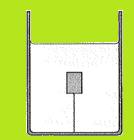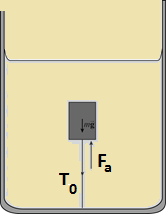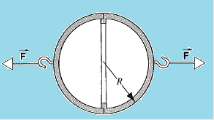Click to Chat

1800-1023-196

+91-120-4616500

CART 0

• 0

MY CART (5)

Use Coupon: CART20 and get 20% off on all online Study Material

ITEM
DETAILS
MRP
DISCOUNT
FINAL PRICE
Total Price: Rs.

There are no items in this cart.
Continue Shopping• Complete JEE Main/Advanced Course and Test Series
• OFFERED PRICE: Rs. 15,900
• View Details

Solved Examples on Deformable Bodies and Elastic Deformation:-

Question 1:-

The tension in a string holding a solid block below the surface of a liquid (of density greater than the solid) is T0 when the containing vessel in the below figure is at rest.

Show that the tension T, when the vessel has an upward vertical acceleration a, is given by T0(1+a/g).Concept:-

The various forces acting on the block when it is inside the water are the weight, the buoyant force and the tension in the string. When the vessel is at rest, there is no net force acting on the block. The sum of the three forces is equal to zero when the vessel is at rest.

When the vessel moves upward with an acceleration, the buoyant force and the tension in the string will be differ. There is a net force acting vessel. Thus, the sum of the three forces is equal to the net force acting on the block.

Solution:-

The weight of the block having mass m which is acting downward is

W = mg

Here,

Acceleration due to gravity at the point of observation is g

The magnitude of the buoyant which acts upwards is

Fb = Vρg

Here,

Volume of the water displaced by the block is V

Density of the water is ρ

The various forces acting on the block which is placed inside the vessel is shown below:As the vessel is rest, the three forces are in equilibrium. Thus, the sum of the three forces is equal to zero.

Thus,

∑F = 0

This makes

W-Fb-T0 = 0

Here,

Tension in the string when the vessel is at rest is T0.

Insert the values of the various terms involved in the above equation gives

Fb-W-T0 = 0

Vρg-mg - T0 = 0

T0 = Vρg-mg

This represents the tension in the string when the vessel is at rest.

When the vessel is moving with an upward vertical acceleration a, there is net forces acting on the block. So, there is change in the buoyant force and the tension in the string.

The buoyant forces acting on the block is

Fb,a = Vρ(g+a)

Thus, the net forces is given as

∑F = ma

It is equal to the sum of the buoyant force, the weight of the block and tension (T).

Thus,

Fb,a-W-T = ma

Substitute the values of Fb,a  and W  in the above equation gives

Fb,a-W-T = ma

Vρ(a+g) – mg - T = ma

T = Vρa+ Vρg -mg-ma

To obtain the tension in the string, substitute T0 = Vρg-mg in the above equation.

T = Vρg - mg + Vρa -ma

= T0 + a (Vρ-m)

Dividing both numerator and denominator in the second part in the right hand side of the equation by g gives

T = T0+[(a/g) (Vρg – mg)]

= T0+[(a/g) T0]

= T0[1+ (a/g)]

This represents the tension in the string when the vessel is accelerating upward.

___________________________________________________________________________________

Question 2:-

Using a soap solution for which the surface tension is 0.025N/m, a child blows a soap bubble of radius 1.40 cm. How much energy is expended in stretching the soap surface?

Concept:-

Surface tension is the surface potential energy per unit area of surface.

The surface area of the soap bubble blown by the child having radius r is

A = 4πr2

The surface tension of the soap bubble is

γ = U/2A

The factor 2 is due to the presence of two surfaces of the soap bubble.

Substitute A =4πr2 in the above equation, the surface tension becomes

γ = U/2A

= U/[2(4πr2)]

= U/8πr2

Solution:-

From the above equation, the energy stored in the surface of the soap bubble is

U = 8γπr2

This much of energy is used in stretching the soap surface.

To obtain required energy, substitute 1.40 cm for the radius r of the soap bubble, 0.025 N/m for the surface tension of the soap bubble γ in the equation U = 8γπr2

U = 8γπr2

= 8(0.025 N/m) (3.14) (1.40 cm)2 (10-2 m/1 cm)2

= (1.2308810-4 N.m) (1 J/1 N.m)

= 1.2308810-4 J

Rounding off to three significant figures, the energy expend in stretching the soap surface is 1.23×10-4 J.

_____________________________________________________________________________________

Question 3:-

In 1654 Otto von Guericke, Burgermeister of Magdeburg and inventor of the air pump, gave a demonstration before the Imperial Diet in which two teams of horses could not pull apart two evacuated brass hemispheres. (a) Show that the force F required to pull apart the hemispheres is F = πR2Δp, where R is the (outside) radius of the hemispheres and Δp is the difference in pressure outside and inside the sphere in the below figure. (b) Taking R equal to 0.305 m and the inside pressure as 0.100 atm, what force would the team of horses have had to exert to pull apart the hemispheres? (c) Why were two teams of horses used? Would not one team prove the point just as well?Concept:-

The two brass hemispheres with an open flat can be replaced with two hemispheres with a closed flat end.

The force required to pull apart the hemispheres is equal to pressure times the surface area.

Solution:-

(a) It is given that the pressure difference between outside and inside the sphere is Δp. The radius of the circle formed by the half hemispheres which face each other is R.

Now, the surface area of the hemisphere is

A = πR2

The force required to pull the apart the brass hemisphere is

F = ΔpA

=Δp(πR2)

= πR2 Δp

Therefore, the force required to pull apart the two hemispheres is πR2Δp.

(b) It is given that the inside pressure is 0.11 atm.

Thus, the pressure difference between the inside and outside of the hemispheres is

Δp = 1.00 atm – 0.11 atm

= 0.89 atm

Substitute 0.305 m for R  and 0.89 atm   for Δp  in the equation F = πR2Δp,

F = πR2Δp

= (3.14) (0.305 m)2 (1.01×105 Pa/1 atm) (1 kg/m.s2/1 Pa)

= (2.5997×104 kg.m/s2) (1 N/1 kg.m/s2)

= 2.5997×104 N

Rounding off to three significant figures, the force required to pull apart the two hemispheres by the team of horses is 2.60×104 N.

(c) The two teams of horses were used so as to pull apart the brass hemispheres. They could not do so as the pressure difference is too high. For such pressure difference, large amount of force is required.### Course Features

• 728 Video Lectures
• Revision Notes
• Previous Year Papers
• Mind Map
• Study Planner
• NCERT Solutions
• Discussion Forum
• Test paper with Video Solution